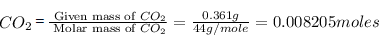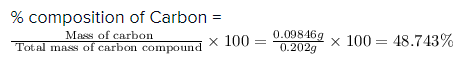Courses

# Atoms And Molecules - Olympiad Level MCQ, Class 9 Science

## 35 Questions MCQ Test Olympiad Preparation for Class 9 | Atoms And Molecules - Olympiad Level MCQ, Class 9 Science

Description
This mock test of Atoms And Molecules - Olympiad Level MCQ, Class 9 Science for Class 9 helps you for every Class 9 entrance exam. This contains 35 Multiple Choice Questions for Class 9 Atoms And Molecules - Olympiad Level MCQ, Class 9 Science (mcq) to study with solutions a complete question bank. The solved questions answers in this Atoms And Molecules - Olympiad Level MCQ, Class 9 Science quiz give you a good mix of easy questions and tough questions. Class 9 students definitely take this Atoms And Molecules - Olympiad Level MCQ, Class 9 Science exercise for a better result in the exam. You can find other Atoms And Molecules - Olympiad Level MCQ, Class 9 Science extra questions, long questions & short questions for Class 9 on EduRev as well by searching above.
QUESTION: 1

Solution:
QUESTION: 2

### The weight of two elements which combine with each other are in the ratio of their :-

Solution:
The equivalent weight of any substance is the mass of the substance in grams that combines with or is chemically equivalent to eight grams of oxygen or one gram of hydrogen. In other words, it is equal to the mass of the substance in grams that would react with or replace one gram of hydrogen. Equivalent weight is expressed as the ratio of atomic weight or molecular weight to the valence.The n number of equivalents is the part of this equation is the quantity of charge in an equation, and what charge is used is depends on the scenario.
QUESTION: 3

### How many moles of oxygen atoms are present in one mole of acetic acid?

Solution: Formula of ACETIC ACID is CH3COOH. so from the chemical formula, you get that number of oxygen atoms in a molecule of acetic acid=2so, 1 mol of acetic acid will contain 2 moles of oxygen atomshope.
QUESTION: 4

What is the number of particles in one mole of a substance?

Solution:
1 mole of any substance contains 6.022 x 1023 particles.
6.022 x 1023 is known as the Avogadro Number or Avogadro Constant and is given the symbol NA(1)
N = n x NA
N = number of particles in the substanc
n = amount of substance in moles (mol)
NA = Avogardro Number = 6.022 x 1023 particles mol-1
N = n x (6.022 x 1023)
To find the number of particles, N, in a substance:
N = n x NA
N = n x (6.022 x 1023)
To find the amount of substance in moles, n :
n = N x NA
n = N x (6.022 x 1023)
QUESTION: 5

How many atoms and how many gram atoms are there in 10 grams of calcium ?

Solution:

No.of particles = mass/ molecular mass x avagadro constant

mass of calcium = 10 g
molecular mass of calcium = 40 u
therefore no of particles = 10/40 x 6.022 x 1023
= 1.5055 x 1023.

=> Gram atoms is mole which is equal to =10/40
=0.25

QUESTION: 6

Calculate the weight of 0.1 mole of sodium carbonate :-

Solution:
moles = mass in gm/mol.wt of compound

0.1 moles of sodium carbonate=wt. of Na2CO3/1O6

wt. of Na2CO3=10.6 gm
QUESTION: 7

How many number of moles are present in 540 g of glucose?

Solution:
Molecular weight of glucose =180g
Thus, 180g =1 mole
So, 540 g= 540/180=3 moles.
QUESTION: 8

Formula for Aluminium Oxide is:

Solution:
QUESTION: 9

Chemical analysis of a carbon compound gave the following percentage composition by weight of the elements
present in it. Carbon = 10.06%, hydrogen = 0.84%, chlorine = 89.10%. Calculate the empirical formula of the compound :-

Solution:
QUESTION: 10

0.202 g of a carbon compound, on combustion, gave 0.361 g of carbon dioxide and 0.47 g of water. Calculate
the percentage composition of carbon :-

Solution:
Given,

Mass of carbon compound = 0.202 g

Mass of carbon dioxide = 0.361 g

Mass of water = 0.47 g

Molar mass of carbon = 12 g/mole

Molar mass of Carbon dioxide = 44 g/mole

First we have to calculate the moles of .

Moles of  =The moles of  = 0.008205 moles

In , there are 1 carbon atom and 2 atom molecules are present.

So, the moles of  is equal to the moles of .

The moles of  = 0.008205 moles

Now we have to calculate the mass of Carbon.

Mass of Carbon = Moles of Carbon X Molar mass of Carbon

Mass of Carbon = 0.008205 moles X 12 g/mole = 0.09846 g

Now we have to calculate the % composition of Carbon.Therefore, the % composition of Carbon is 48.743%.
QUESTION: 11

One mole of CO2 contains :

Solution:
QUESTION: 12

The percentage of nitrogen in ammonia is given by the expression :-

Solution:
QUESTION: 13

The empricial formula of a compound is CH2O. Its molecular weight is 90. Calculate the molecular formula of
the compound. (Atomic weight C = 12, H = 1, O = 16)

Solution:
QUESTION: 14

Calculate the weight of 2.5 mole of CaCO3 :-

Solution:
QUESTION: 15

How many gram atoms are present in 256 g of O2 ?

Solution:
QUESTION: 16

The molecular formula of potassium nitrate is ________.

Solution:
QUESTION: 17

The number of moles present in 20 grams of CaCO3 is :-

Solution:

No, i think the answer is no. of moles =actual mass/ molecular weight no. of moles=20/41+12+16*3 no. of moles=20/101 no. of moles=0.19801

QUESTION: 18

A hydrogen contain 90% of carbon and 10% hydrogen. The empirical formula of the compound is :-

Solution:
QUESTION: 19

An element has only one type of :-

Solution:
QUESTION: 20

The simplest formula of a compound having 50% of X(atomic weight 10) and 50% of Y(atomic weight 20) is :-

Solution:
QUESTION: 21

How many moles of glucose (C6H12O6) are present in 5.4 g ?

Solution:

molecular wt of glucose C6H12O6

6*12+12*1+6*16

72+12+96

180

no of moles = wt/mol wt

=5.4/180

=54/1800

=27/900

=9/300

=3/100

=0.03

QUESTION: 22

Calculate the number of gram atoms present in 8g of helium

Solution:
QUESTION: 23

How many moles are present in 5.3 g of anhydrous sodium carbonate ?

Solution:
Moles of Na2CO3=5.3/106=0.05
so moles of Na=0.05x2=0.1
moles of C=0.05x1=0.05
moles of O=0.05x3=0.15
we can now find the no. of atoms of each type by multiplying the no. of moles by Avagadro's constant.

QUESTION: 24

Calculate the number of moles in 60 gram NaOH.

Solution: According to me, no. of moles = given mass/molecular mass no. of moles = 60/23 +16+ 1 no. of moles = 60/40 no. of moles = 3/2 no. of moles = 1.5
QUESTION: 25

Calculate the weight of nitrogen present in 0.5 moles of NH3.

Solution:
QUESTION: 26

Calculate the number of moles present in 7.3 g of HCl.

Solution:
QUESTION: 27

Calculate the weight in gram of 0.9 gram atoms of zinc.

Solution:
QUESTION: 28

Calculate the weight of 0.4 gram atoms of carbon

Solution:
QUESTION: 29

What is the weight of 3-gram atoms of sulphur?

Solution:

Weight of atom = atomic mass × Given mass

⇒ 3g × 32g

= 96 g

weight of 3 grams of atoms of sulphur is 96 g.

QUESTION: 30

16 gram of oxygen is equal to :-

Solution:
QUESTION: 31

When a neutral atom is converted into anion its :-

Solution:
QUESTION: 32

Which of the following is an ionic compound ?

Solution:
QUESTION: 33

The bond formed between two atoms of an electronegative element is :-

Solution:
QUESTION: 34

Ionic compounds :

Solution:
QUESTION: 35

Covalent compound commonly exhibit :-

Solution: